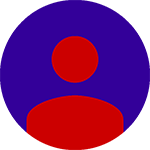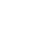• No products in the basket.

# Percentage Test Question with Solution – TNPSC Aptitude Video Class

## Test Question With Solution – Percentage

1.50 kg is what percent of 250 kg?

a) 26%      b) 15%      c)20%      d) 18%

## Solution

Here Percentage is asked, so, Formula 1

(50/250) x 100

= 20%

2.If 20% of x= 90, find x.

a) 350        b) 450       c) 250       d) 550

## Solution

Here percentage is given,

20% of x = 90

x X (20/100) = 90

x = (90 x 100)/20

x = 450

3. What is 5% of 50% of 500?

a) 12.5       b) 25      c) 1.25      d)6.25

## Solution

5 % of 50 % of 500

Let us take : 50 % of 500

then [500 x(50/100)]

Now, 5% of [500 x(50/100)]

Therefore, [500 x (50/100)] x (5/100) = 25/2

=12.5

4. A person’s salary has increased from Rs. 7200 to 8100. what is the percentage increase in salary?

a) 25 %        b)18 %         c)16 2/3 %       d)12  1/2 %

## Solution

% change = (change in value / initial value) x 100

change in value = 8100 – 7200

= 900

% change = (900/7200) x 100

=12.5

5. The strength of a college was 3000 last year. If there is an increase of 20 % this year the the present strength is

a) 3200         b) 3400       c) 3600       d) 3800

## Solution

Strength = 3000

increment = 3000 x (20/100)

= 600

present strength = 3000 + 600

= 3600

6. In a company, there are 724 employees. 25 % employees left the job. Find the number of rest of employees.

a) 543       b) 550       c) 578      d) 565

## Solution

Total employees = 724

25 % of 724 = 724 x (25/100)

= 181

181 employees left the company.

therefore, 724 – 181 = 543

7. The population of a town is 126800 increases by 15% in the first year and decreases by 20 % in the second year. What is the population of the town at the end of second year?

a) 174984       b) 135996        c) 116656      d) 145820

8) The length of a rectangle is increased by 50%. By what percent would the breadth have to be decreased to maintain the same area?

a) 37.5 %             b) 33.33 %           c) 25 %            d) None of these.

## Solution

area of rectangle = L x B

let initial L = 100% B = 100%

So, area = 100 x 100 = 10000

length is increased by 50 %, New length = 150

area = 150 x b = 10000 (same area)

b = 10000/150

b = 66.66 %

Question is By what % breadth have to be decreased?

So, % change = (change in value)/(Initial Value x 100)

= [(100 – 66.66%)/100] x 100

= 33.33 %

9) Find the percentage increase in the area of a triangle if its each side is doubled.

a) 100 %             b) 200 %           c) 300 %            d) 400 %

## Solution

area = 1/2 bh

If sides are doubled, then b = 2b; h = 2h

Then, area = 1/2 (2b x 2h)

= 2bh

% difference = ((Final value – Initial Value)/Initial Value ) X 100

= ((2bh – 0.5 bh) / 0.5 bh) X 100

= 1.5 x 2 x 100

= 300 %

10) Ramesh spent 20% of his pocket money on buying clothes. After spending 25% of the remainder in buying shoes,  he has Rs.480 left. What was his pocket money?

a) Rs.600             b) Rs.800           c) Rs.900            d) Rs.1000

## Solution

Let the total pocket money be Y.

Rupees spent on clothes is Y * 20/100

The remaining amount after spending on clothes is,

Y  –  (20Y/100)  ;    [  Y -> Total ;      (20Y/100) -> Clothes ]

=  (100Y – 20Y)/100

= 80Y/100

25 % of the above remainder is spent on shoes, therefore

25 % of (80Y/100)  =   (25/100) * (80Y/100)

= (80Y * 25) / 10000

= 2000 Y / 10000

Therefore, Total Money Y = (20Y/100) + (2000Y/10000) + 480

(clothes)      +    (shoes)                + (remaining left)

Y = (2000Y  +   2000 Y  +  4800000 ) / 10000

Y = (4000 Y + 4800000 ) / 10000

10000 Y = 4000 Y + 4800000

10000 Y – 4000 Y = 4800000

6000 Y = 4800000

Y = 4800000 / 6000

Y = 800 rupees

## Watch TNPSC Aptitude video class in Tamil & English – Percentage

### 17 responses on "Percentage Test Question with Solution - TNPSC Aptitude Video Class"

1.9th n 10th question solutions plz

•Will update soon.

•9th and 10th solutions updated.

2.Thank u 🙂

•You are welcome.

3.pls upload the video of profit and loss aptitude sir

4.is tr any shrt ct for 10th qtn

5.really it is useful to me and very easy to understand thank a lot sir

•You are welcome.

6.I did understand one problem from your practice type question while checking the answer in the time.

Ramesh spent 20% of pocket mny on buy clothes. After spending 25% if remainder shoes, he has rs. 480 left. What was his pocket money.

•1. 25% he spend for shoes.
let 100%= 25% + 480 ie( 480=75%)
so 25%= 480/3= 160
then 100%= 160+480 = 640
2. 20% he spend for cloths
let 100%= 20%+ 640(640= 80%)
so 20%= 160 (i.e 640/4)
so finally the 100% = 160+640=800
hope u got it ????

7.Very easy to understand.

8.I see you don’t monetize your blog, don’t waste your traffic, you
can earn additional bucks every month because you’ve got hi quality content.
If you want to know how to make extra money, search for:
Ercannou’s essential tools best adsense alternative

9.I have checked your site and i have found some duplicate content, that’s why you don’t rank
high in google’s search results, but there is a
tool that can help you to create 100% unique articles, search for; Boorfe’s
tips unlimited content

10. 1 2
Scan for APPLandmark: Opposite to M.S.Mahal,
Chennai – 600014.

PH: 7904003050